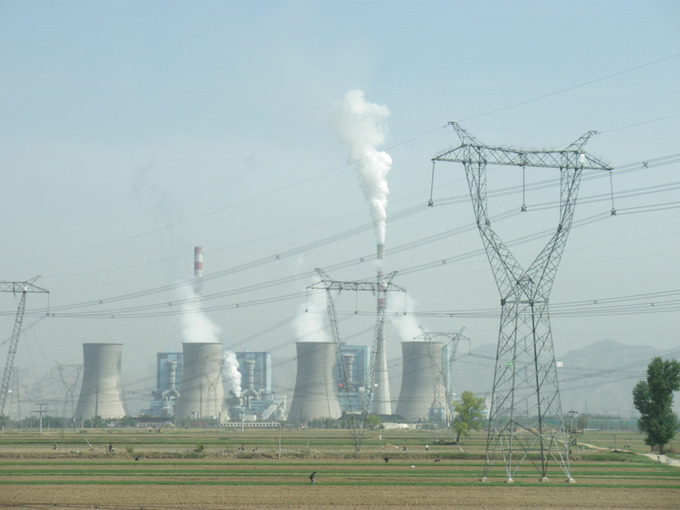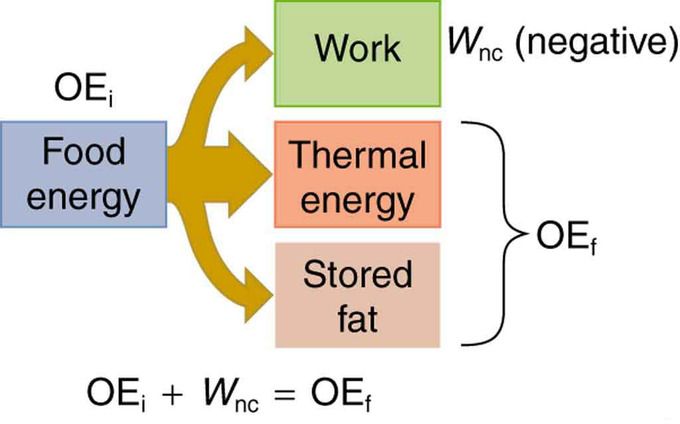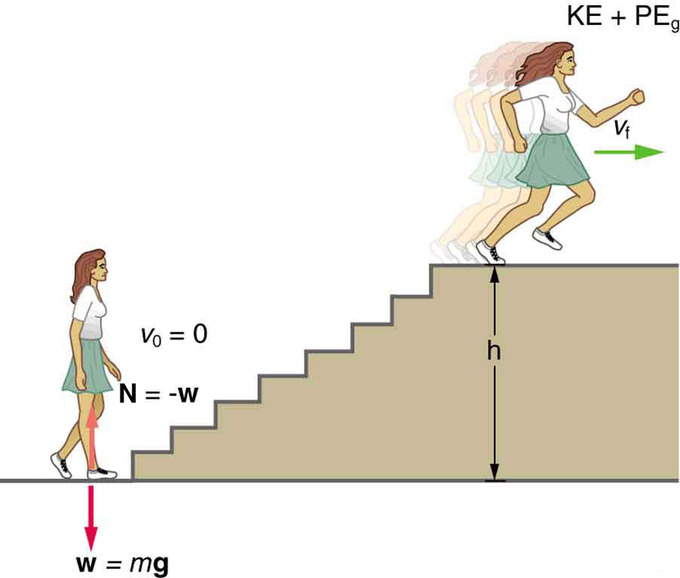# What is Power?

In physics, power is the rate of doing work—the amount of energy consumed per unit time.

### Learning Objectives

Relate power to the transfer, use, and transformation of different types of energy

### Key Takeaways

#### Key Points

• Power implies that energy is transferred, perhaps changing form.
• Energy transfer can be used to do work, so power is also the rate at which this work is performed.
• The unit of power is the joule per second (J/s), known as the watt.

#### Key Terms

• power: A measure of the rate of doing work or transferring energy.
• watt: In the International System of Units, the derived unit of power; the power of a system in which one joule of energy is transferred per second.

In physics, power is the rate of doing work. It is the amount of energy consumed per unit time. The unit of power is the joule per second (J/s), known as the watt (in honor of James Watt, the eighteenth-century developer of the steam engine). For example, the rate at which a lightbulb transforms electrical energy into heat and light is measured in watts (W)—the more wattage, the more power, or equivalently the more electrical energy is used per unit time.

Power: A brief overview of power in an algebra-based physics course.

Energy transfer can be used to do work, so power is also the rate at which this work is performed. The same amount of work is done when carrying a load up a flight of stairs whether the person carrying it walks or runs, but more power is expended during the running because the work is done in a shorter amount of time. The output power of an electric motor is the product of the torque the motor generates and the angular velocity of its output shaft. The power expended to move a vehicle is the product of the traction force of the wheels and the velocity of the vehicle.

Examples of power are limited only by the imagination, because there are as many types as there are forms of work and energy. Sunlight reaching Earth’s surface carries a maximum power of about 1.3 kilowatts per square meter (kW/m2). A tiny fraction of this is retained by Earth over the long term. Our consumption rate of fossil fuels is far greater than the rate at which they are stored, so it is inevitable that they will be depleted. Power implies that energy is transferred, perhaps changing form. It is never possible to change one form completely into another without losing some of it as thermal energy. For example, a 60-W incandescent bulb converts only 5 W of electrical power to light, with 55 W dissipating into thermal energy. Furthermore, the typical electric power plant converts only 35 to 40 percent of its fuel into electricity. The remainder becomes a huge amount of thermal energy that must be dispersed as heat transfer, as rapidly as it is created. A coal-fired power plant may produce 1,000 megawatts; 1 megawatt (MW) is 106 W of electric power. But the power plant consumes chemical energy at a rate of about 2,500 MW, creating heat transfer to the surroundings at a rate of 1,500 MW.Coal-fired Power Plant: Tremendous amounts of electric power are generated by coal-fired power plants such as this one in China, but an even larger amount of power goes into heat transfer to the surroundings. The large cooling towers here are needed to transfer heat as rapidly as it is produced. The transfer of heat is not unique to coal plants but is an unavoidable consequence of generating electric power from any fuel—nuclear, coal, oil, natural gas, or the like.

## Humans: Work, Energy, and Power

The human body converts energy stored in food into work, thermal energy, and/or chemical energy that is stored in fatty tissue.

### Learning Objectives

Identify what factors play a role in basal metabolic rate (BMR)

### Key Takeaways

#### Key Points

• The rate at which the body uses food energy to sustain life and to do different activities is called the metabolic rate, and the corresponding rate when at rest is called the basal metabolic rate (BMR).
• The energy included in the basal metabolic rate is divided among various systems in the body, with the largest fraction going to the liver and spleen, and the brain coming next.
• About 75% of food calories are used to sustain basic body functions included in the basal metabolic rate.
• Work done by a person is sometimes called useful work, which is work done on the outside world, such as lifting weights.
• The energy consumption of people during various activities can be determined by measuring their oxygen use, because the digestive process is basically one of oxidizing food.

#### Key Terms

• basal metabolic rate: The amount of energy expended while at rest in a neutrally temperate environment, in the post-absorptive state.

### Humans: Work, Energy, and Power

Our own bodies, like all living organisms, are energy conversion machines. Conservation of energy implies that the chemical energy stored in food is converted into work, thermal energy, or stored as chemical energy in fatty tissue, as shown in. Energy consumed by humans is converted to work, thermal energy, and stored fat. By far the largest fraction goes to thermal energy, although the fraction varies depending on the type of physical activity. The fraction going into each form depends both on how much we eat and on our level of physical activity. If we eat more than is needed to do work and stay warm, the remainder goes into body fat.Energy Conversion in Humans: Energy consumed by humans is converted to work, thermal energy, and stored fat. By far the largest fraction goes to thermal energy, although the fraction varies depending on the type of physical activity.

### Functions that Require Energy

All bodily functions, from thinking to lifting weights, require energy. The many small muscle actions accompanying all quiet activity, from sleeping to head scratching, ultimately become thermal energy, as do less visible muscle actions by the heart, lungs, and digestive tract. Shivering, in fact, is an involuntary response to low body temperature that pits muscles against one another to produce thermal energy in the body (and do no work). The kidneys and liver consume a surprising amount of energy, but the biggest surprise of all it that a full 25% of all energy consumed by the body is used to maintain electrical potentials in all living cells. (Nerve cells use this electrical potential in nerve impulses. ) This bioelectrical energy ultimately becomes mostly thermal energy, but some is utilized to power chemical processes such as in the kidneys and liver, and in fat production.

### Basal Metabolic Rate

The rate at which the body uses food energy to sustain life and to do different activities is called the metabolic rate. The total energy conversion rate of a person at rest is called the basal metabolic rate (BMR) and is divided among various systems in the body. The largest fraction goes to the liver and spleen, with the brain coming next. Of course, during vigorous exercise, the energy consumption of the skeletal muscles and heart increase markedly. About 75% of the calories burned in a day go into these basic functions. The BMR is a function of age, gender, total body weight, and amount of muscle mass (which burns more calories than body fat). Athletes have a greater BMR due to this last factor.

### Useful Work

Work done by a person is sometimes called useful work, which is work done on the outside world, such as lifting weights. Useful work requires a force exerted through a distance on the outside world, and so it excludes internal work, such as that done by the heart when pumping blood. Useful work does include that done in climbing stairs or accelerating to a full run, because these are accomplished by exerting forces on the outside world. Forces exerted by the body are nonconservative, so that they can change the mechanical energy (KE+PE) of the system worked upon, and this is often the goal.

For example, what is the power output for a 60.0-kg woman who runs up a 3.00 m high flight of stairs in 3.50 s, starting from rest but having a final speed of 2.00 m/s?.Woman Running Up Stairs: When this woman runs upstairs starting from rest, she converts the chemical energy originally from food into kinetic energy and gravitational potential energy. Her power output depends on how fast she does this.

Her power output depends on how fast she does this. The work going into mechanical energy is W= KE + PE. At the bottom of the stairs, we take both KE and PEg as initially zero; thus,

$\text{W} = \text{KE}_\text{f} + \text{PE}_\text{g} = \frac{1}{2}\text{m} \text{v}_\text{f}^2+\text{mgh}$

where h is the vertical height of the stairs. Because all terms are given, we can calculate W and then divide it by time to get power. Substituting the expression for W into the definition of power given in the previous equation, P=W/t yields

$\text{P}=\frac{\text{W}}{\text{t}}=\frac{\frac{1}{2} \text{m} \text{v}_\text{f}^2+\text{mgh}}{\text{t}}$.

Entering known values yields

$\begin{eqnarray} \text{P} &=& \frac{0.5(60.0 \mathrm{\text{kg}})(2.00 \mathrm{\text{m}/\text{s}})^2+(60.0 \mathrm{\text{kg}})(9.80 \mathrm{\text{m}/\text{s}}^2)(3.00 \mathrm{\text{m}})}{(3.50 \mathrm{\text{s}})} \ &=& \frac{120 \mathrm{\text{J}} + 1764 \mathrm{\text{J}}}{3.50 \mathrm{\text{s}}} \ &=& 538 \mathrm{\text{W}} \end{eqnarray}$

The woman does 1764 J of work to move up the stairs compared with only 120 J to increase her kinetic energy; thus, most of her power output is required for climbing rather than accelerating.

Energy consumption is directly proportional to oxygen consumption because the digestive process is basically one of oxidizing food. We can measure the energy people use during various activities by measuring their oxygen use. Approximately 20 kJ of energy are produced for each liter of oxygen consumed, independent of the type of food.